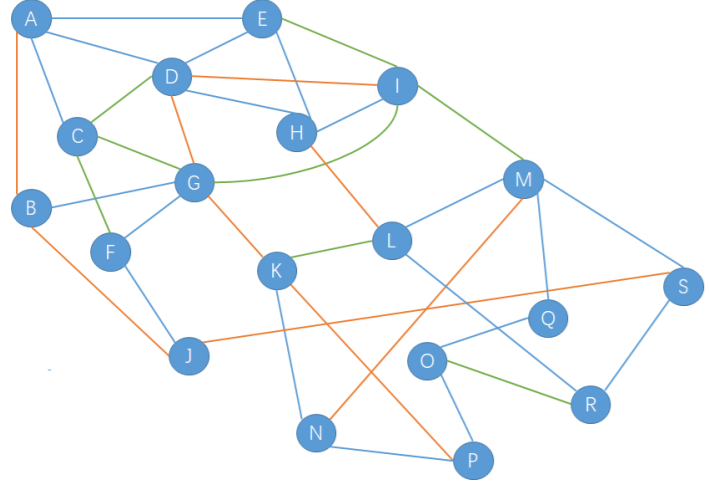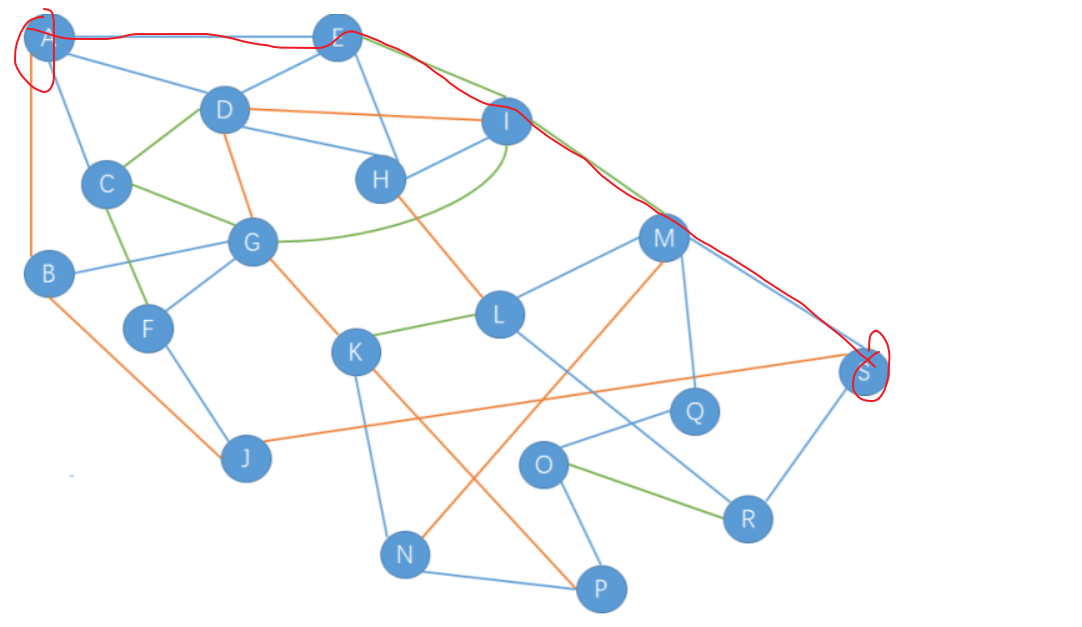### 这里写目录标题

import os
import sys

list = *200
st = input()
for i in st:
column = ord(i)
list[column] = list[column] + 1
print(chr(list.index(max(list))))
print(max(list))



Notes：
1. input()：输入字符串等
2. ord()：字符转ASCII码
3. list = 200：初始化数组
4. chr()：ASCII码转字符
5. max()：搜索最大list最大数值
6. 列表.index()：找出括号内的索引

## 成绩统计

import os
import sys

s = int(input())
p = 0
e =0

for i in range(s):
score = int(input())
if 60 <= score:
p = p +1
if 85 <= score <= 100:
e = e+1

print('{:.0%}'.format(p/s))
print('{:.0%}'.format(e/s))


Notes:
1. int(input())：从键盘输入整型。先input()再int()也是没问题的。
2. range()：range() 函数返回的是一个可迭代对象（类型是对象）详细解释
3. {:.0%}：python3输出百分比详细解释

## 最短路Notes：
1. 这是一道填空题，灵活变通很重要
2. 解决无向图最短路径的问题用Dijkstra算法，Dijkstra算法原理
3. 在这道题里，不建议使用Dijkstra算法计算全部节点，中间那一团太浪费时间了。
4. 我是取巧了，从下图直接算出来了，肉眼就只能看出这条最短，其实可以暴力解决这题，能找到更小的就舍弃小的就可以了。浪费太多时间在填空题不太值这个分值的。## 回文日期

Notes：

1. 公历每年可以分为平年和非闰年，平年和闰年区别在于2月，闰年2月29天 ，平年2月28天。
2. 闰年共有366天（1月~12月分别为31天、29天、31天、30天、31天、30天、31天、31天、30天、31天、30天、31天）。
3. 平年共有365天（1月~12月分别为31天、28天、31天、30天、31天、30天、31天、31天、30天、31天、30天、31天）。
4. 速记口诀：1月大，2月小，3月大，4月小，5月大，6月小，7月大，8月大，9月小，10月大，11月小，12月大。
5. 1582年以来的置闰规则：普通闰年：公历年份是4的倍数，且不是100的倍数的（如2004年、2020年等就是闰年）。世纪闰年：公历年份是整百数的，必须是400的倍数才是闰年（如1900年不是闰年，2000年是闰年）。更多详情
6. 判断闰年需满足 “普通闰年”和“世纪闰年”。

7. 下面答案不全对，没有参考价值。

import os
import sys

# 普通回文数
h1 = 0
# ABABBABA回文数
h2 = 0

# 普通回文数
h3 = 0
# ABABBABA回文数
h4 = 0

time = input()
list = [31,29,31,30,31,30,31,31,30,31,30,31]

year = int(time+time+time+time)
month = int(time+time)
day = int(time+time)
if ((int(time) % 4 == 0 and int(time) % 100 !=0) or (int(time) % 400 ==0)):
list = 28

if int(time) != int(time+time+time+time+time+time+time+time):
if month == int(time+time):
if day <= int(time+time) <= list[month]:
if int(time+time) == int(time+time):
h2 = 1
h4 = int(time+time+time+time+time+time+time+time)
else :
h1 = 1
h3 = int(time+time+time+time+time+time+time+time)
if month < int(time+time) <= 12:
if int(time+time) <= list[month]:
if int(time+time) == int(time+time):
h2 = 1
h4 = int(time+time+time+time+time+time+time+time)
else :
h1 = 1
h3 = int(time+time+time+time+time+time+time+time)
# print(h1,h2,h3,h4)

if not h1 or not h2:
y = year
while not h1 or not h2:
y = int(y)
y = y + 1
list = 29
if (y % 4 == 0 and y % 100 !=0) or (y % 400 ==0):
list = 28
y = str(y)
if not h1:
if int(y + y) != int(y + y):
if 0 < int(y + y) <= 12:
if 0 < int(y + y) <= list[int(y + y) - 1]:
h1 = 1
h3 = int(y + y + y + y + y + y + y + y)
# print(h1, h3)
if not h2:
# print(y)
if int(y + y) == int(y + y):
if 0 < int(y + y) <= 12:
if 0 < int(y + y) <= list[int(y + y) - 1] and int(y + y) == int(y + y):
h2 = 1
h4 = int(y + y + y + y + y + y + y + y)
# print(h2, h4)
if h3 > int(time):
print(h3)
else:
print()
if h4 > int(time):
print(h4)
else:
print()



## 购物单

import os
import sys

print(180.90 * 0.88 +
10.25 * 0.65 +
56.14 * 0.9 +
104.65 * 0.9 +
100.30 * 0.88 +
297.15 * 0.5 +
26.75 * 0.65 +
130.62 * 0.5 +
240.28 * 0.58 +
270.62 * 0.8 +
115.87 * 0.88 +
247.34 * 0.95 +
73.21 * 0.9 +
101.00 * 0.5 +
79.54 * 0.5 +
278.44 * 0.7 +
199.26 * 0.5 +
12.97 * 0.9 +
166.30 * 0.78 +
125.50 * 0.58 +
84.98 * 0.9 +
113.35 * 0.68 +
166.57 * 0.5 +
42.56 * 0.9 +
81.90 * 0.95 +
131.78 * 0.8 +
255.89 * 0.78 +
109.17 * 0.9 +
146.69 * 0.68 +
139.33  * 0.65 +
141.16 * 0.78 +
154.74 * 0.8 +
59.42 * 0.8 +
85.44 * 0.68 +
293.70 * 0.88 +
261.79 * 0.65 +
11.30 * 0.88 +
268.27 * 0.58 +
128.29 * 0.88 +
251.03 * 0.8 +
208.39 * 0.75 +
128.88 * 0.75 +
62.06 * 0.9 +
225.87 * 0.75 +
12.89 * 0.75 +
34.28 * 0.75 +
62.16 * 0.58 +
129.12 * 0.5 +
218.37 * 0.5 +
289.69 * 0.8)
# print('5200')


## 递增序列

LANN
QIAO

VLPWJVVNNZSWFGHSFRBCOIJTPYNEURPIGKQGPSXUGNELGRVZAG
SDLLOVGRTWEYZKKXNKIRWGZWXWRHKXFASATDWZAPZRNHTNNGQF
BDGMGYHAOPPRRHKYZCMFZEDELCALTBSWNTAODXYVHQNDASUFRL
YVYWQZUTEPFSFXLTZBMBQETXGXFUEBHGMJKBPNIHMYOELYZIKH
ZYZHSLTCGNANNXTUJGBYKUOJMGOGRDPKEUGVHNZJZHDUNRERBU
XFPTZKTPVQPJEMBHNTUBSMIYEGXNWQSBZMHMDRZZMJPZQTCWLR
ZNXOKBITTPSHEXWHZXFLWEMPZTBVNKNYSHCIQRIKQHFRAYWOPG
VIDPIGELBYMEVQLASLQRUKMXSEWGHRSFVXOMHSJWWXHIBCGVIF
GWRFRFLHAMYWYZOIQODBIHHRIIMWJWJGYPFAHZZWJKRGOISUJC
PEKNJFIVBKOZUEPPHIWLUBFUDWPIDRJKAZVJKPBRHCRMGNMFWW
CGZAXHXPDELTACGUWBXWNNZNDQYYCIQRJCULIEBQBLLMJEUSZP
RWHHQMBIJWTQPUFNAESPZHAQARNIDUCRYQAZMNVRVZUJOZUDGS
PFGAYBDEECHUXFUZIKAXYDFWJNSAOPJYWUIEJSCORRBVQHCHMR
JNVIPVEMQSHCCAXMWEFSYIGFPIXNIDXOTXTNBCHSHUZGKXFECL
YZBAIIOTWLREPZISBGJLQDALKZUKEQMKLDIPXJEPENEIPWFDLP
HBQKWJFLSEXVILKYPNSWUZLDCRTAYUUPEITQJEITZRQMMAQNLN
DQDJGOWMBFKAIGWEAJOISPFPLULIWVVALLIIHBGEZLGRHRCKGF
LXYPCVPNUKSWCCGXEYTEBAWRLWDWNHHNNNWQNIIBUCGUJYMRYW
CZDKISKUSBPFHVGSAVJBDMNPSDKFRXVVPLVAQUGVUJEXSZFGFQ
IYIJGISUANRAXTGQLAVFMQTICKQAHLEBGHAVOVVPEXIMLFWIYI
HGTEEIISFGIUEUOWXVTPJDVACYQYFQUCXOXOSSMXLZDQESHXKP
FEBZHJAGIFGXSMRDKGONGELOALLSYDVILRWAPXXBPOOSWZNEAS
VJGMAOFLGYIFLJTEKDNIWHJAABCASFMAKIENSYIZZSLRSUIPCJ
BMQGMPDRCPGWKTPLOTAINXZAAJWCPUJHPOUYWNWHZAKCDMZDSR
RRARTVHZYYCEDXJQNQAINQVDJCZCZLCQWQQIKUYMYMOVMNCBVY


NOTES：
1. 注意45°和135°的区别，题目只要45°方向的，不要135°方向的。
2. python存储多端字符串的手段：

b = 'abc'
d = 'def'
a = [] # 定义空列表
a.append(b) # a = [‘abc’]
a.append(d) # a = [‘abc’, ‘def’]
# 如果要调用d的值,可以通过列表下标索引，如a 为‘abc’，a为‘a’。


3. 要注意的是，一条线上的两个字母！45°一条线上的容易遗忘。

import os
import sys

list = ['VLPWJVVNNZSWFGHSFRBCOIJTPYNEURPIGKQGPSXUGNELGRVZAG',
'SDLLOVGRTWEYZKKXNKIRWGZWXWRHKXFASATDWZAPZRNHTNNGQF',
'BDGMGYHAOPPRRHKYZCMFZEDELCALTBSWNTAODXYVHQNDASUFRL',
'YVYWQZUTEPFSFXLTZBMBQETXGXFUEBHGMJKBPNIHMYOELYZIKH',
'ZYZHSLTCGNANNXTUJGBYKUOJMGOGRDPKEUGVHNZJZHDUNRERBU',
'XFPTZKTPVQPJEMBHNTUBSMIYEGXNWQSBZMHMDRZZMJPZQTCWLR',
'ZNXOKBITTPSHEXWHZXFLWEMPZTBVNKNYSHCIQRIKQHFRAYWOPG',
'VIDPIGELBYMEVQLASLQRUKMXSEWGHRSFVXOMHSJWWXHIBCGVIF',
'GWRFRFLHAMYWYZOIQODBIHHRIIMWJWJGYPFAHZZWJKRGOISUJC',
'PEKNJFIVBKOZUEPPHIWLUBFUDWPIDRJKAZVJKPBRHCRMGNMFWW',
'CGZAXHXPDELTACGUWBXWNNZNDQYYCIQRJCULIEBQBLLMJEUSZP',
'RWHHQMBIJWTQPUFNAESPZHAQARNIDUCRYQAZMNVRVZUJOZUDGS',
'PFGAYBDEECHUXFUZIKAXYDFWJNSAOPJYWUIEJSCORRBVQHCHMR',
'JNVIPVEMQSHCCAXMWEFSYIGFPIXNIDXOTXTNBCHSHUZGKXFECL',
'YZBAIIOTWLREPZISBGJLQDALKZUKEQMKLDIPXJEPENEIPWFDLP',
'HBQKWJFLSEXVILKYPNSWUZLDCRTAYUUPEITQJEITZRQMMAQNLN',
'DQDJGOWMBFKAIGWEAJOISPFPLULIWVVALLIIHBGEZLGRHRCKGF',
'LXYPCVPNUKSWCCGXEYTEBAWRLWDWNHHNNNWQNIIBUCGUJYMRYW',
'CZDKISKUSBPFHVGSAVJBDMNPSDKFRXVVPLVAQUGVUJEXSZFGFQ',
'IYIJGISUANRAXTGQLAVFMQTICKQAHLEBGHAVOVVPEXIMLFWIYI',
'HGTEEIISFGIUEUOWXVTPJDVACYQYFQUCXOXOSSMXLZDQESHXKP',
'FEBZHJAGIFGXSMRDKGONGELOALLSYDVILRWAPXXBPOOSWZNEAS',
'VJGMAOFLGYIFLJTEKDNIWHJAABCASFMAKIENSYIZZSLRSUIPCJ',
'BMQGMPDRCPGWKTPLOTAINXZAAJWCPUJHPOUYWNWHZAKCDMZDSR',
'RRARTVHZYYCEDXJQNQAINQVDJCZCZLCQWQQIKUYMYMOVMNCBVY',
ans = 0

for x in range(0,30):
for y in range(0,50):
m = y
n = x
for w in range(m,50): # 列
if ord(list[x][y]) < ord(list[x][w]):
ans = ans + 1
for w in range(n,30): # 行
if ord(list[x][y]) < ord(list[w][y]):
ans = ans + 1
for i in range(0,50): # 从左下角到右上角和从右上角到左下角
if 0 <= x - i < 30 and 0 <= y + i < 50:
if ord(list[x - i][y + i]) != ord(list[x][y]):
ans = ans + 1
if 0 <= x + i < 30 and 0 <= y + i < 50: # 从左上角到右下角
if ord(list[x + i][y + i]) > ord(list[x][y]):
ans = ans + 1
print(ans)


## 排序

Notes：

1. 了解冒泡排序原理（这是原理
2. 题目要求（1）最短字符串（2）最小字典序（3）100次交换
3. 完全逆序交换次数最多，字符串最短。
公式：完全逆序交换次数 = n * ( n - 1 ) / 2，n为字符个数。
套公式求得最小字符串长度为15，最小完全逆序交换次数为105
4. 为满足“最小字典序”，a~o15个字符。
5. a交换15次，b交换14次，c交换13次，以此类推，将第6个（j）往前交换5次，换到o前面。即jonmlkihgfedcba

## 成绩分析

Notes：

1. 保留两位小数’{:.2f}’.format()。

import os
import sys

MAX = 0
MIN = 100
SUM = 0
nums = int(input())
for i in range(0,nums):
L = int(input())
SUM = SUM + L
if MAX < L:
MAX = L
if MIN > L:
MIN = L
print(MAX)
print(MIN)
print('{:.2f}'.format(SUM/nums))


## 棋盘放麦子

import os
import sys

num = 1
nums = 1
for i in range(1,64):
num = num*2
nums = nums + num
print(nums)



## 长草

4 5
.g...
.....
..g..
.....
2


gggg.
gggg.
ggggg
.ggg.


Notes：

1. 了解BFS（宽搜）原理及过程并应用宽搜原理
2. 了解python输入多个数字以空格隔开
3. 了解python如何输入二维数组每行
4. 了解python分别输出二维数组每行
import os
import sys

# 输入行n和列m
n,m = map(int,input().split())
# 创建一个一维数组，用于存储初始化后的草坪中的草的坐标
lawn = []
# 创建一个队列，用于存储未访问过的草的坐标
queue = []
# 循环输入每行
for i in range(n):
lawn.append(list(map(str,input())))
# 存储每行草的坐标
for j in range(len(lawn[i])):
if lawn[i][j] == 'g':
queue.append([i,j])

# 输入多少个月
k = int(input())

if k == 0:
for l in lawn:
for row in l:
print(row,end='\n')

# 循环每个月草生长情况
for month in range(k):
lens = len(queue)
for q in range(lens):
# 取队列队首
temp = queue.pop(0)

# 草左边的格子
x = temp
y = temp-1
if 0 <= y < m and lawn[x][y] != 'g':
queue.append([x,y])
lawn[x][y] = 'g'

# 草右边的格子
y = temp+1
if 0 <= y < m and lawn[x][y] != 'g':
queue.append([x,y])
lawn[x][y] = 'g'

# 草下方的格子
x = temp+1
y = temp
if 0 <= x < n and lawn[x][y] != 'g':
queue.append([x,y])
lawn[x][y] = 'g'

# 草上方的格子
x = temp-1
if 0 <= x < n and lawn[x][y] != 'g':
queue.append([x,y])
lawn[x][y] = 'g'

for i in range(n):
for j in range(m):
print(lawn[i][j],end='')
print()


## 寻扎2020

1. 同一行里面连续四个字符从左到右构成 2020。
2. 同一列里面连续四个字符从上到下构成 2020。
3. 在一条从左上到右下的斜线上连续四个字符，从左上到右下构成 2020。
这是数据

Notes
1. 暴力枚举

import os
import sys

# 数据格式
list = ['123','456','……',]
ans = 0
rows = len(list)
cols = len(list)
# print(row,col)
for i in range(rows):
for j in range(cols):

if j+3<rows:
if int(str(list[i][j])+str(list[i][j+1])+str(list[i][j+2])+str(list[i][j+3])) == 2020:
ans +=1
if i+3<cols:
if int(str(list[i][j])+str(list[i+1][j])+str(list[i+2][j])+str(list[i+3][j])) == 2020:
ans +=1
if i+3<rows and j+3<cols:
if int(str(list[i][j]) + str(list[i + 1][j+1]) + str(list[i + 2][j+2]) + str(list[i + 3][j+3])) == 2020:
ans +=1
print(ans)
# 16520



## 全球变暖

.......
.##....
.##....
....##.
..####.
...###.
.......


.......
.......
.......
.......
....#..
.......
.......


7
.......
.##....
.##....
....##.
..####.
...###.
.......


1

Notes：

1. 利用BFS宽搜的思想求解宽搜
import os
import sys

# 海域长宽
n = int(input())
# 存储字符
sea = []
# 标记有不会被淹没的小岛
land = 0
# 小岛数量
island = 0
# 没有被淹没的小岛数量
count = 0
# 创建队列
queue = []
# 输入每行字符
for _ in range(n):
sea.append(list(map(str,input())))

for i in range(n):
for j in range(n):
# 初始化为0，表示已淹没
land = 0
# 找到一个小岛
if sea[i][j] == '#':
island += 1
queue.append((i,j))
# 已访问过的赋值为*
sea[i][j] = '*'
# 循环一个小岛上所有#
while queue:
# 取队首
a,b = queue.pop(0)
# 循环上下左右是否为#
for x,y in [(0,-1),(0,1),(-1,0),(1,0)]:
temp_i = a + x
temp_j = b + y
if 0 <= temp_i < n and 0 <= temp_j < n:
if sea[temp_i][temp_j] == '#':
# 周围没有.，表示此小岛没有被淹没
if (0 <= temp_j - 1 < n and sea[temp_i][temp_j-1] != '.') and (0 <= temp_j + 1 < n and sea[temp_i][temp_j+1] != '.') and (0 <= temp_i - 1 < n and sea[temp_i-1][temp_j] != '.') and (0 <= temp_i + 1 < n and sea[temp_i+1][temp_j] != '.'):
land = 1
queue.append((temp_i,temp_j))
sea[temp_i][temp_j] = '*'
# 没有被淹没的小岛数量加1
if land == 1:
count += 1
print(island-count)